# Chapter 24 Model Building

Author: Ron Reviewer:

## 24.1 Introduction

### 24.1.1 prerequisites

``````library(tidyverse)
library(modelr)
options(na.action = na.warn)

library(nycflights13)
library(lubridate)``````

## 24.2 Why are low quality diamonds more expensive

### 24.2.1 Price and carat

Note:

Residuals of a model contain the information that doesn’t get caught by this model. By examining the residual of the following model

``fit1 <- lm(data = diamonds,log(price)~log(carat))``

A positive relationship of the residual with one additional predictor means there is a positive correlation between them. For example, `residual ~ cut` means cut may partially explain the increase of the prediction error of `fit1`. This becomes more obvious when the residual is transformed back from `log` scale which explains the exponential price growth with respect to `cut` or `clarity`.

### 24.2.3 Exercises

1.In the plot of `lcarat` vs. `lprice`, there are some bright vertical strips. What do they represent?

``````diamonds2 <- diamonds %>%
filter(carat <= 2.5) %>%
mutate(lprice = log2(price), lcarat = log2(carat))

ggplot(diamonds2, aes(lcarat, lprice)) +
geom_hex(bins = 50)``````
``````## Warning: Computation failed in `stat_binhex()`:
## Package `hexbin` required for `stat_binhex`.
## Please install and try again.``````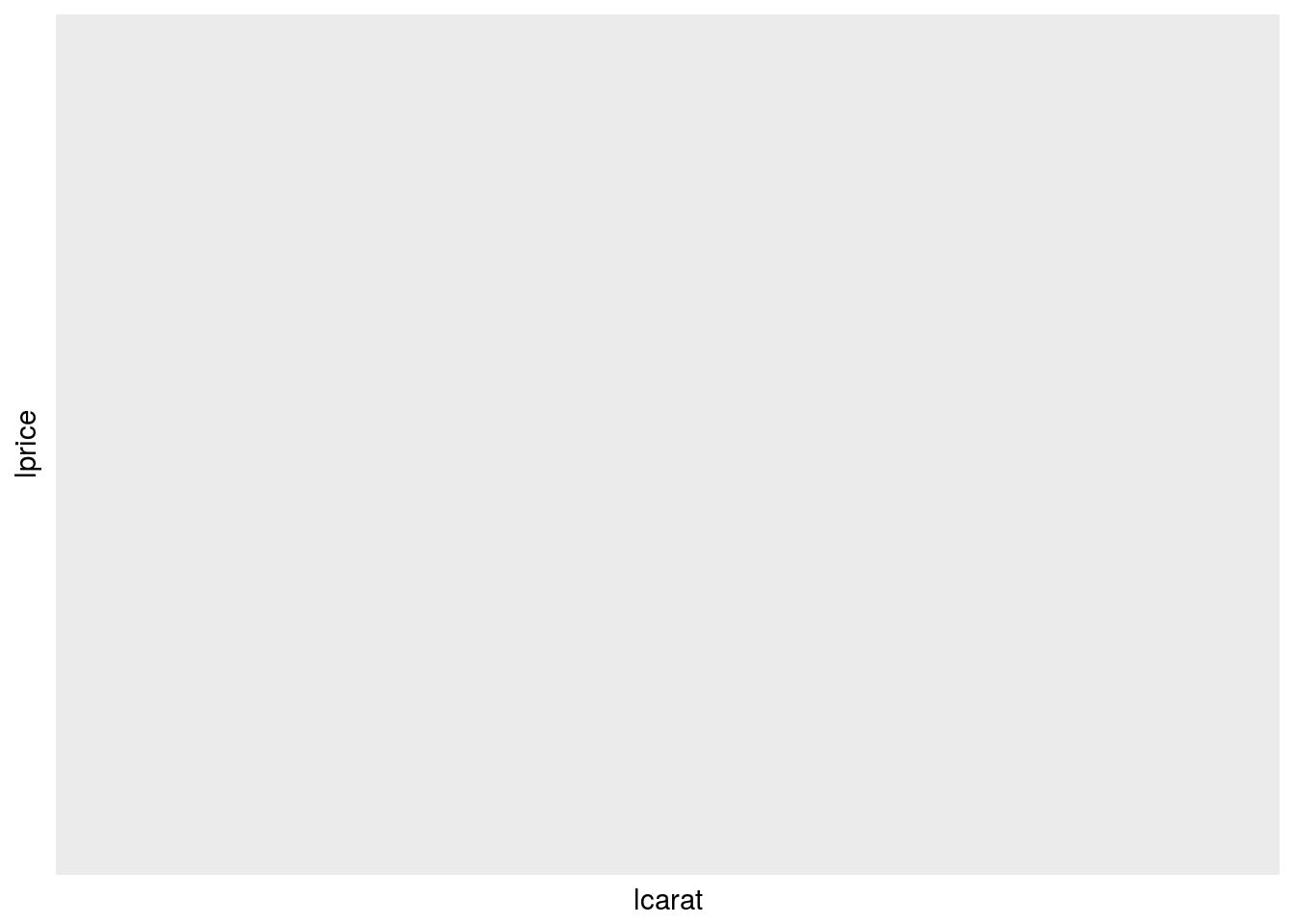Those bright vertical strips mean that many diamonds are cut to those “standard” or popular “weight” since bright means higher count.

2.If `log(price) = a_0 + a_1 * log(carat)`, what does that say about the relationship between price and carat?

It means `price = exp(a_0)*carat^(a_1)`.

3.Extract the diamonds that have very high and very low residuals. Is there anything unusual about these diamonds? Are the particularly bad or good, or do you think these are pricing errors?

``````diamonds2 <- diamonds %>%
filter(carat <= 2.5) %>%
mutate(lprice = log2(price), lcarat = log2(carat))

mod_diamond <- lm(lprice ~ lcarat, data = diamonds2)

diamonds2 <- diamonds2 %>%

summary(diamonds2\$lresid)``````
``````##      Min.   1st Qu.    Median      Mean   3rd Qu.      Max.
## -1.964068 -0.245488 -0.008442  0.000000  0.239301  1.934855``````
``````diamonds3 <- diamonds2 %>% filter(lresid > quantile(lresid)[] | lresid < quantile(lresid)[] )

table(diamonds3\$cut)``````
``````##
##      Fair      Good Very Good   Premium     Ideal
##       317      1651      5515      6477     12946``````
``table(diamonds3\$clarity)``
``````##
##   I1  SI2  SI1  VS2  VS1 VVS2 VVS1   IF
##    1  836 4321 7340 5304 4208 3225 1671``````
``````diamonds3 %>%
ggplot(aes(clarity,price))+
geom_boxplot()+
facet_grid(~cut)``````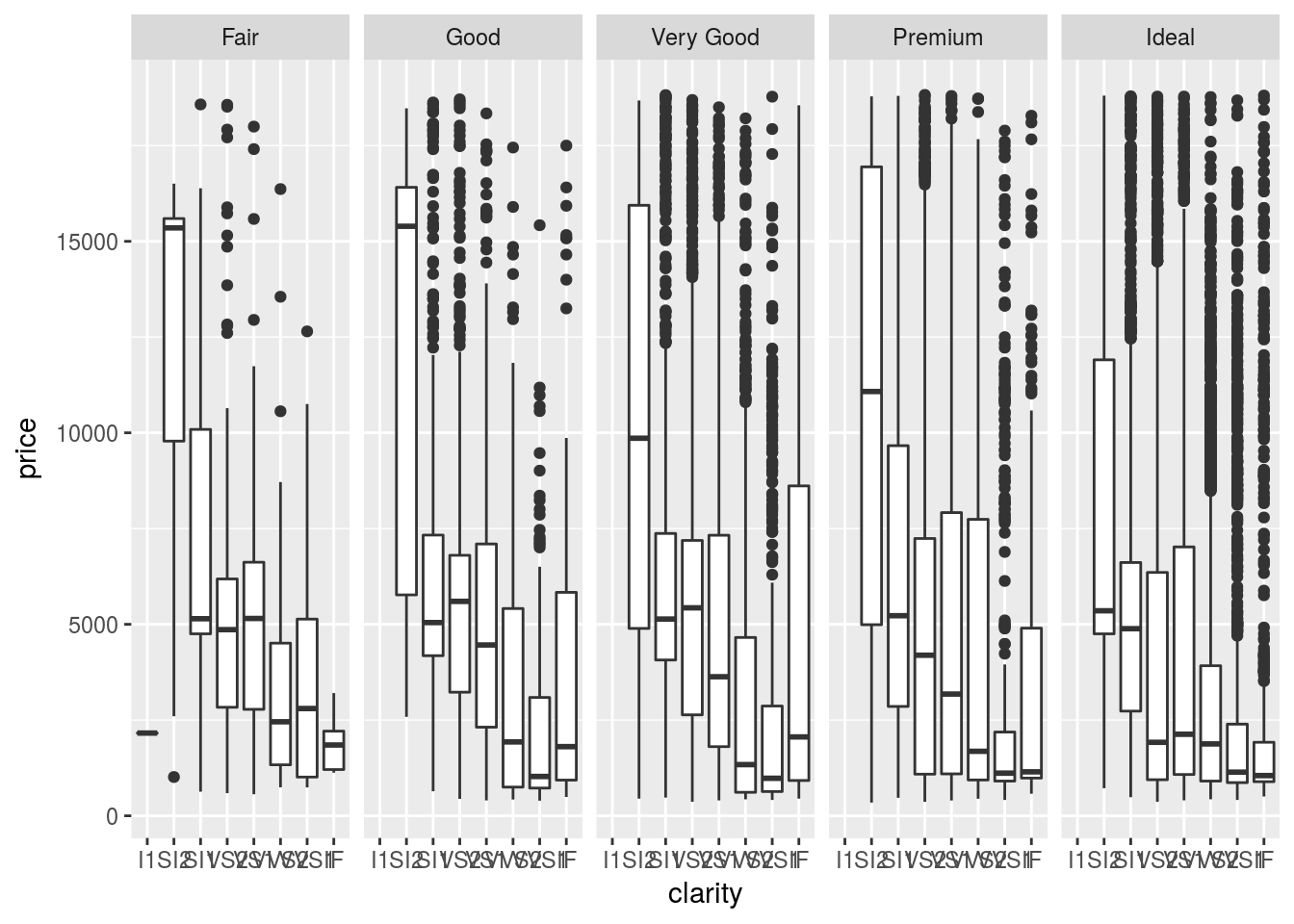There are surely errrs here. diamonds with better clarity are priced lower.

1. No. Since the predictors are not normalized and treated equally in the model. There are some diamonds which are very expensive but predicted to be cheap or vice versa.

## 24.3 What affects the number of daily flights

### 24.3.1 Day of week

Some useful functions from package `lubridate`

``````# make date
lubridate::make_date(1992,8,12)``````
``##  "1992-08-12"``
``````# find weekday
lubridate::make_date(1992,8,12) %>%
lubridate::wday(label = TRUE)``````
``````##  Wed
## Levels: Sun < Mon < Tue < Wed < Thu < Fri < Sat``````
``````# make date as year month day
lubridate::ymd(20130101)``````
``##  "2013-01-01"``

### 24.3.2 Seasonal Saturday effect

``````daily <- flights %>%
mutate(date = make_date(year, month, day)) %>%
group_by(date) %>%
summarise(n = n())
daily <- daily %>%
mutate(wday = wday(date, label = TRUE))``````
``````daily %>%
filter(wday == "Sat") %>%
ggplot(aes(date, n)) +
geom_point() +
geom_line() +
scale_x_date(NULL, date_breaks = "1 month", date_labels = "%b") # set x tick as a month``````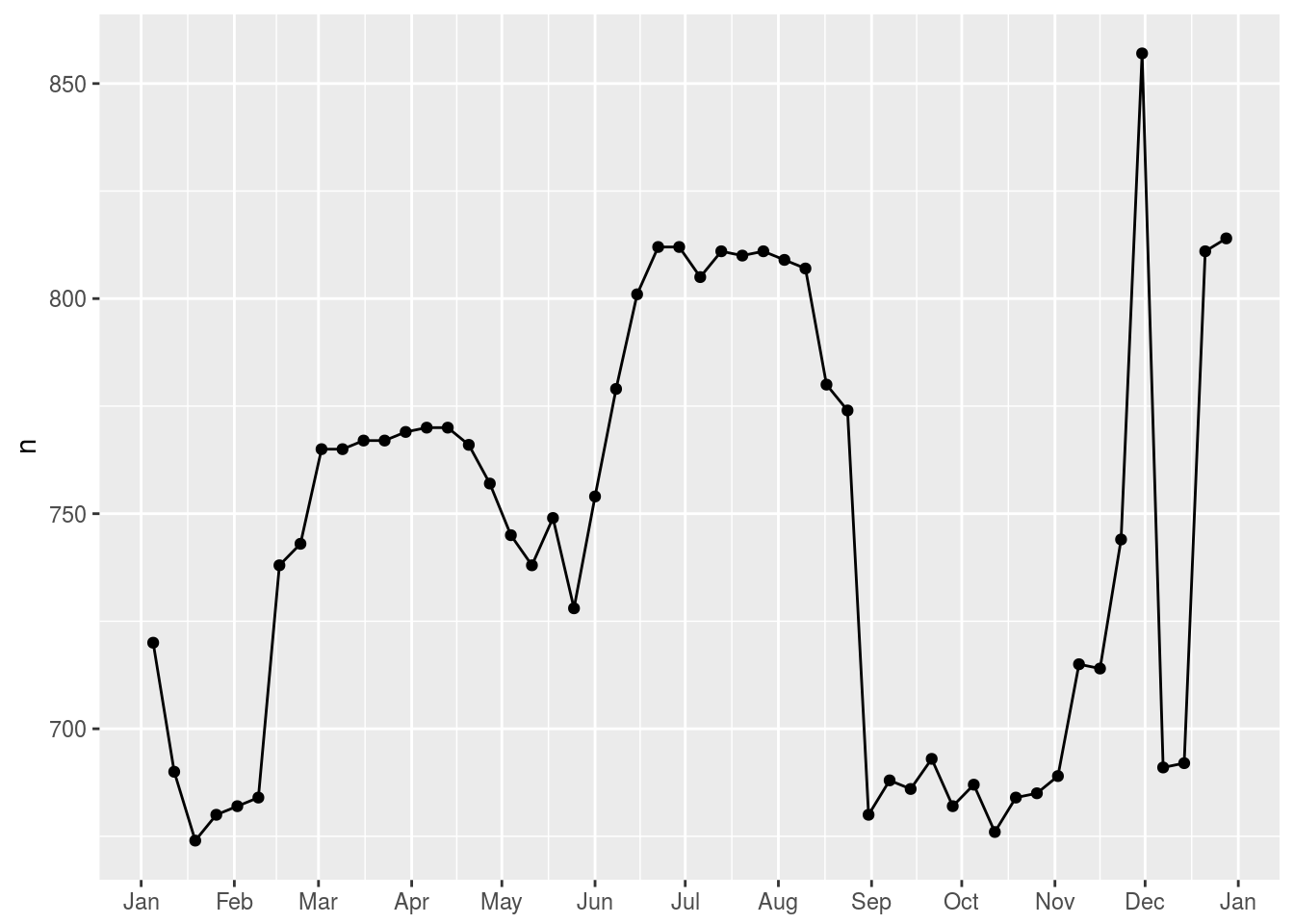### 24.3.3 Computed variables

Note:

It is preferred to make computed/transformed variables explicit for further visualization purpose if memory permits.

### 24.3.5 Exercises

1. Use your Google sleuthing skills to brainstorm why there were fewer than expected flights on Jan 20, May 26, and Sep 1. (Hint: they all have the same explanation.) How would these days generalise to another year?

They are close to the Martin Luther King day, the trinity Sunday and the Labor day. They can’t be generalised to other years because those dates depend on weekdays.

Check the website.

1. What do the three days with high positive residuals represent? How would these days generalise to another year?
``````# repeat what is done in the book
term <- function(date) {
cut(date,
breaks = ymd(20130101, 20130605, 20130825, 20140101),
labels = c("spring", "summer", "fall")
)
}

daily <- daily %>%
mutate(term = term(date))

daily %>%
filter(wday == "Sat") %>%
ggplot(aes(date, n, colour = term)) +
geom_point(alpha = 1/3) +
geom_line() +
scale_x_date(NULL, date_breaks = "1 month", date_labels = "%b")``````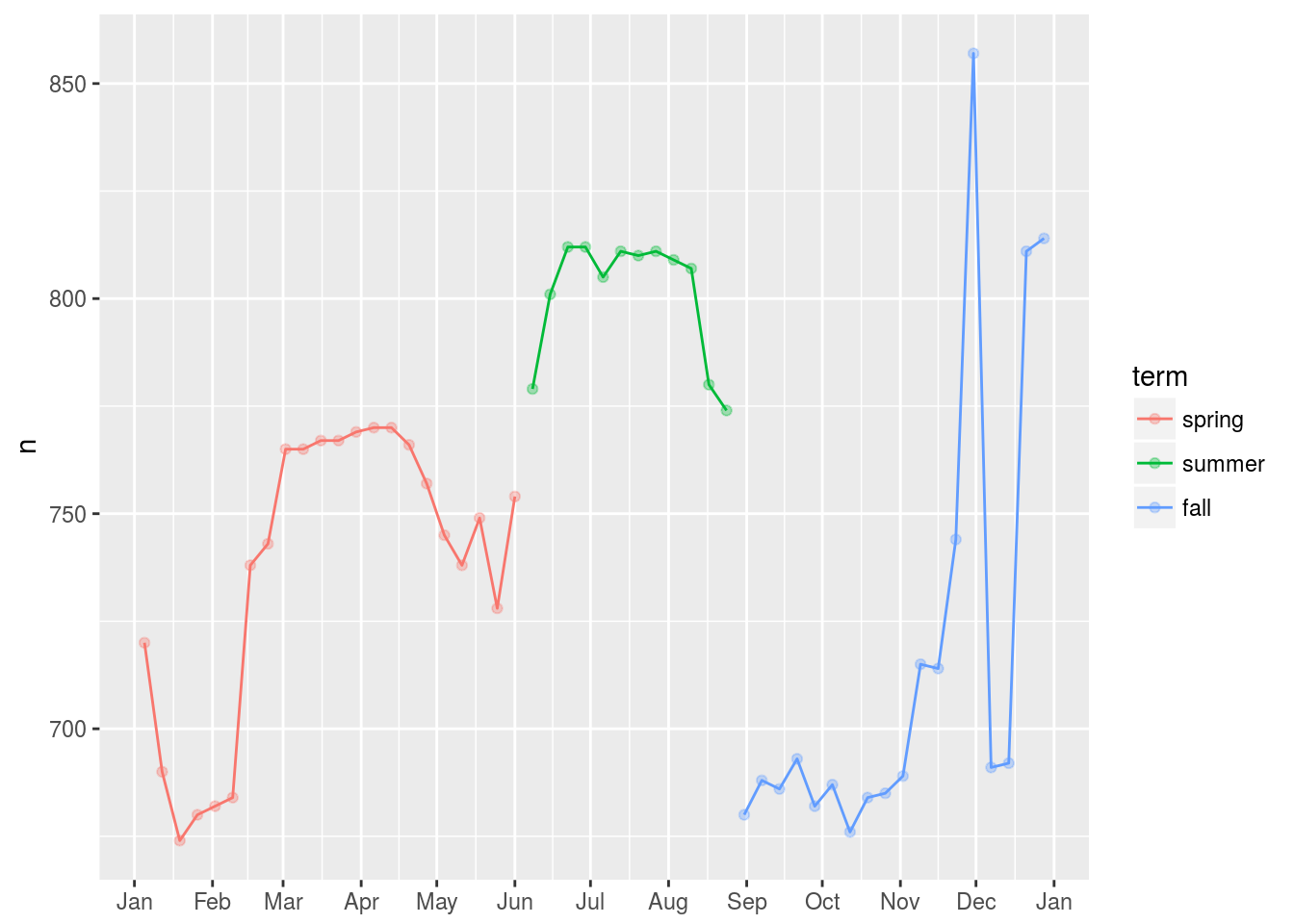``````mod1 <- lm(n ~ wday, data = daily)
mod2 <- lm(n ~ wday * term, data = daily)

daily %>%
gather_residuals(without_term = mod1, with_term = mod2) %>%
ggplot(aes(date, resid),color = model) +
geom_line(alpha = 0.75) +
facet_grid(~model)``````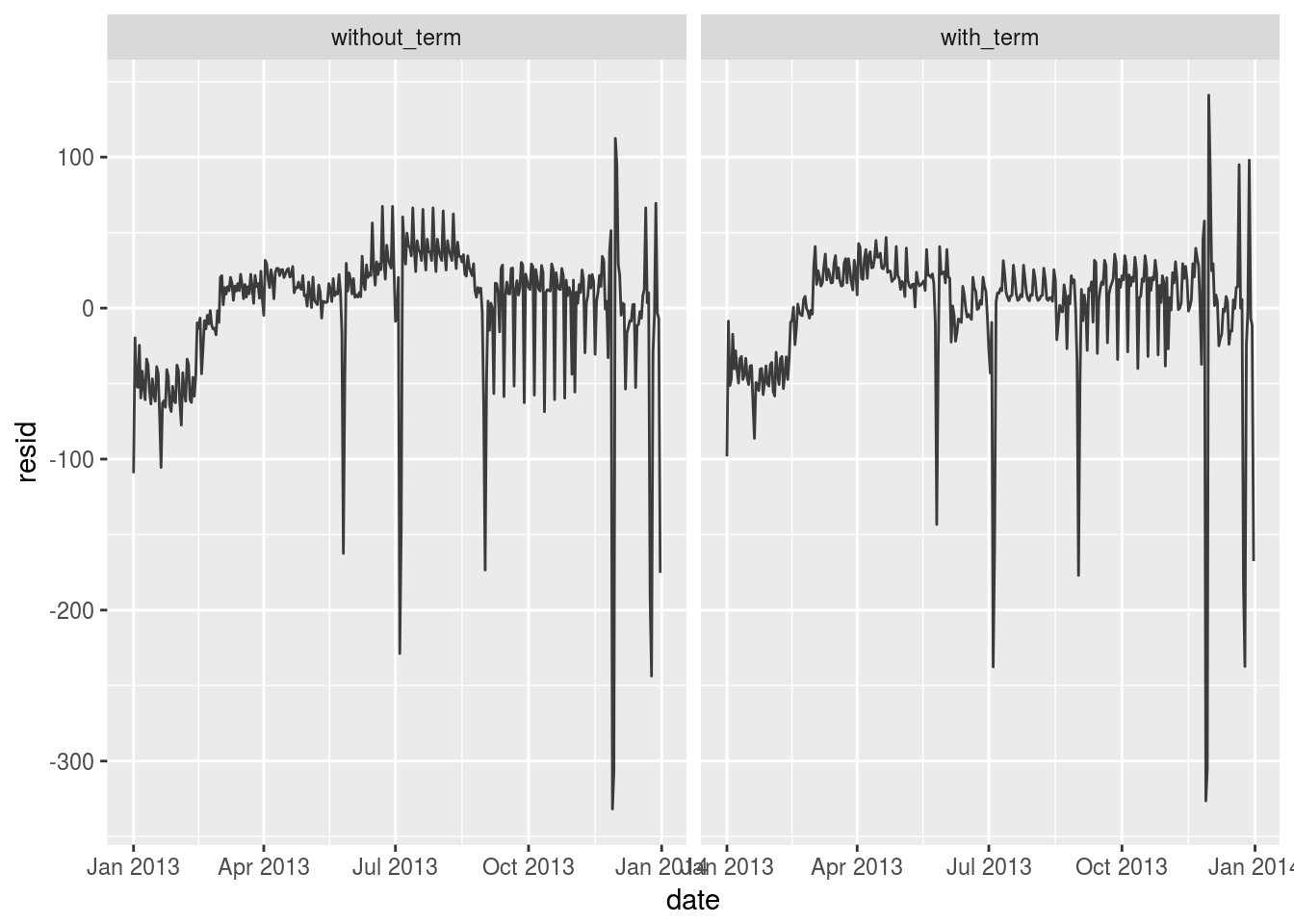``````daily %>%
top_n(3, resid)``````
``````## # A tibble: 3 x 6
##         date     n  wday   term     resid     pred
##       <date> <int> <ord> <fctr>     <dbl>    <dbl>
## 1 2013-11-30   857   Sat   fall 112.38462 744.6154
## 2 2013-12-01   987   Sun   fall  95.51923 891.4808
## 3 2013-12-28   814   Sat   fall  69.38462 744.6154``````

Those dates are close to Thanks giving and New Year. The travel need is high.

1. Create a new variable that splits the wday variable into terms, but only for Saturdays, i.e. it should have `Thurs`, `Fri`, but `Sat-summer`, `Sat-spring`, `Sat-fall`. How does this model compare with the model with every combination of `wday` and `term`?
``````daily <- daily %>%
mutate(term = term(date))  %>%
mutate(term2 = ifelse(wday == 'Sat',paste0(wday,"-",term),as.character(term) ))

mod3 <- lm(n~ wday * term2, data = daily)

daily %>%
gather_residuals(mod3,mod2)%>%
arrange(date)%>%
ggplot(aes(date,resid,color = model))+
geom_line(alpha = 0.75)``````
``````## Warning in predict.lm(model, data): prediction from a rank-deficient fitWe get a warning saying that `prediction from a rank-deficient fit may be misleading`. This is understandable since our `term2` variable is constructed from `term` and `wday`. The prediction result is exactly the same.

1. Create a new `wday` variable that combines the day of week, term (for Saturdays), and public holidays. What do the residuals of that model look like? chron package has a function called `is.holiday` Requires additonal work to identify American Public holidays
``# to do``

5.What happens if you fit a day of week effect that varies by month (i.e. `n ~ wday * month`)? Why is this not very helpful?

``````daily <- flights %>%
mutate(date = make_date(year, month, day)) %>%
group_by(date,month) %>%
summarise(n = n())
daily <- daily %>%
mutate(wday = wday(date, label = TRUE)) %>%
mutate(term = term(date))
mod2 <- lm(n ~ wday * term, data = daily)
mod4 <- lm(n~ wday * month, data = daily)

daily %>%
gather_residuals(mod4,mod2)%>%
arrange(date)%>%
ggplot(aes(date,resid,color = model))+
geom_line(alpha = 0.75)``````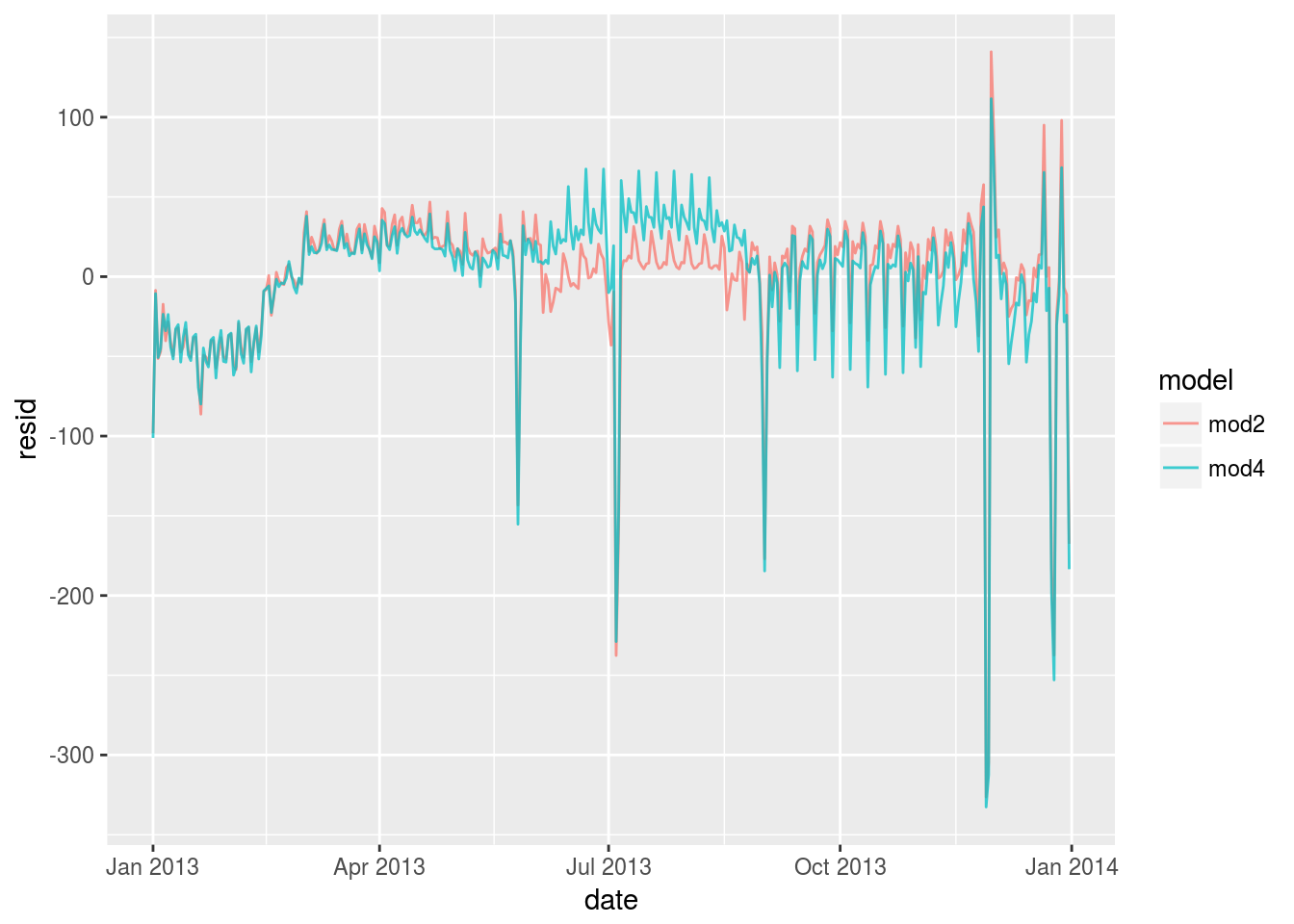It can be understood this way that `month` information is part of the `date` information so the performance will be actually a little bit worse.

1. What would you expect the model `n ~ wday + ns(date, 5)` to look like? Knowing what you know about the data, why would you expect it to be not particularly effective?
``````mod5 <- lm(n ~ wday + splines::ns(date, 5), data = daily)
daily %>%
gather_residuals(mod2,mod5)%>%
arrange(date)%>%
ggplot(aes(date,resid,color = model))+
geom_line(alpha = 0.75)``````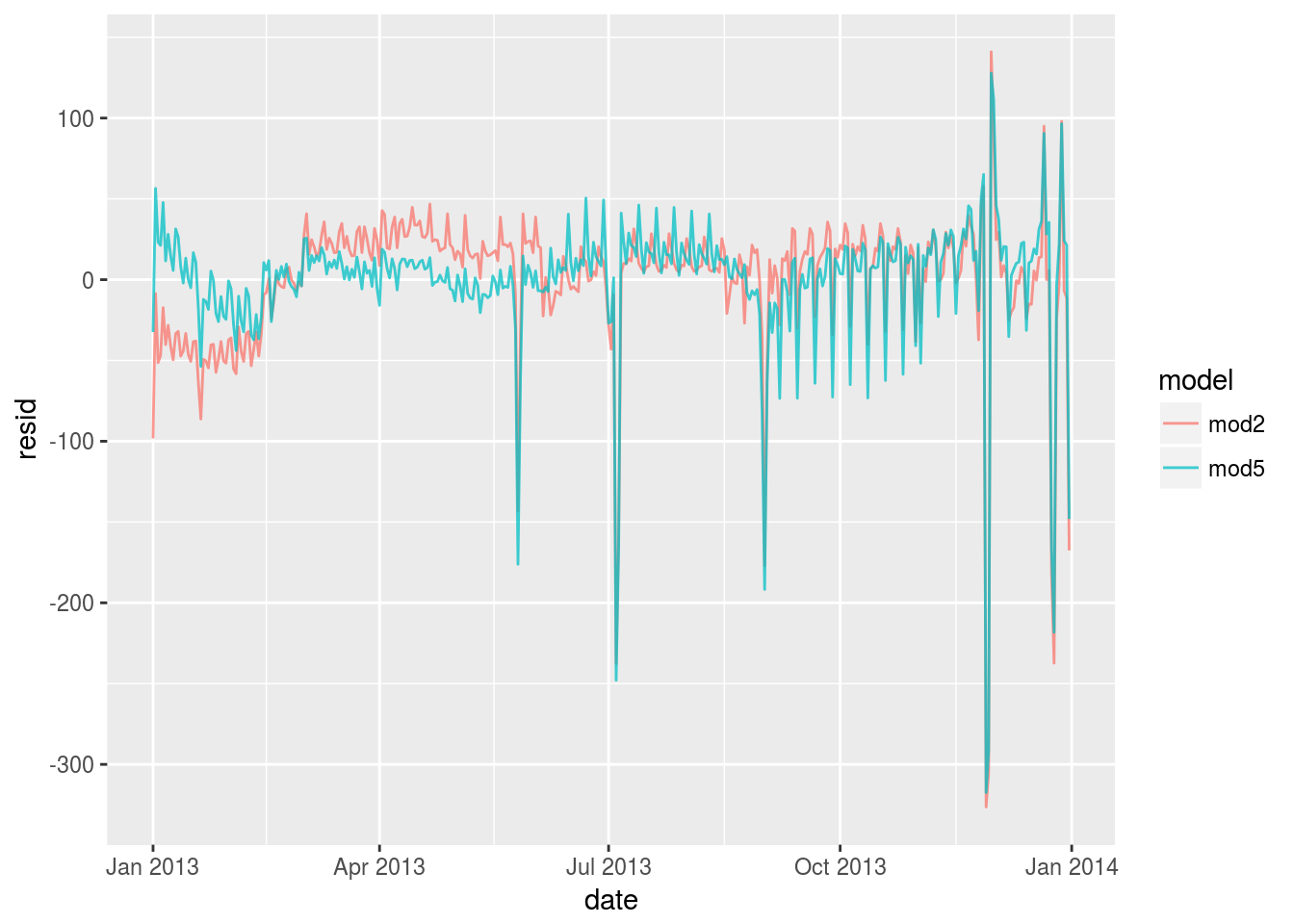Splines does better job when `n` changes slowly but it has a systematic overestimation in the fall semester. It shouldn’t be particularly effective.

1. We hypothesised that people leaving on Sundays are more likely to be business travellers who need to be somewhere on Monday. Explore that hypothesis by seeing how it breaks down based on distance and time: if it’s true, you’d expect to see more Sunday evening flights to places that are far away.
``````dist <- function(distance) {
cut(distance,
breaks = seq(min(flights\$distance,na.rm = TRUE),max(flights\$distance,na.rm = TRUE),length.out = 10),
labels = letters[1:9]
)
}

time <- function(air_time){
cut(air_time,
breaks = seq(min(flights\$air_time,na.rm = TRUE),max(flights\$air_time,na.rm = TRUE),length.out = 10),
labels = letters[1:9]
)
}

dep <- function(dep_time){
cut(dep_time,
breaks = seq(0000,2400,length.out = 9),
labels = c("0-3","3-6","6-9","9-12","12-15","15-18","18-21","21-24")
)
}

flights <- flights %>%
mutate(dist = dist(distance)) %>%
mutate(time = time(air_time)) %>%
mutate(dep = dep(dep_time))%>%
mutate(wday= wday(make_date(year, month, day), label = TRUE))``````

Visualize the flights distance distribution

``````flights %>%
ggplot() +
geom_bar(aes(dep,color = dist),position = "dodge") +
facet_wrap(~wday, nrow=3)``````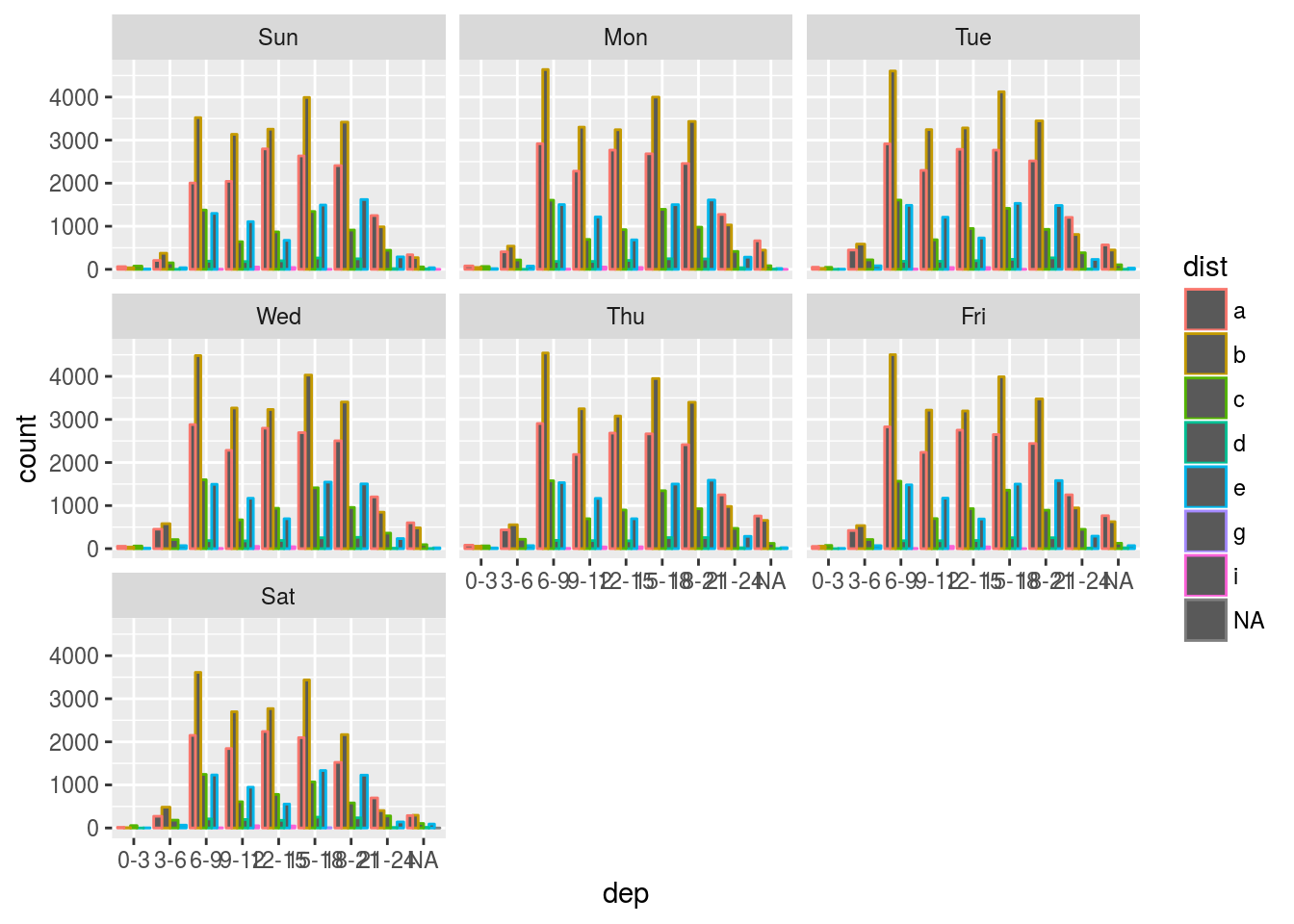As far as the graph explains, the hypothesis is not true.

8.It’s a little frustrating that Sunday and Saturday are on separate ends of the plot. Write a small function to set the levels of the factor so that the week starts on Monday.

Reorder the factor wday.

``flights <- rbind(flights %>% filter(wday != 'Sun'),flights %>% filter(wday == 'Sun'))``

## 24.4 Learning more about models

1. `Statistical Modeling: A Fresh Approach`
2. `An Introduction to Statistical Learning`
3. `Applied Predictive Modeling`